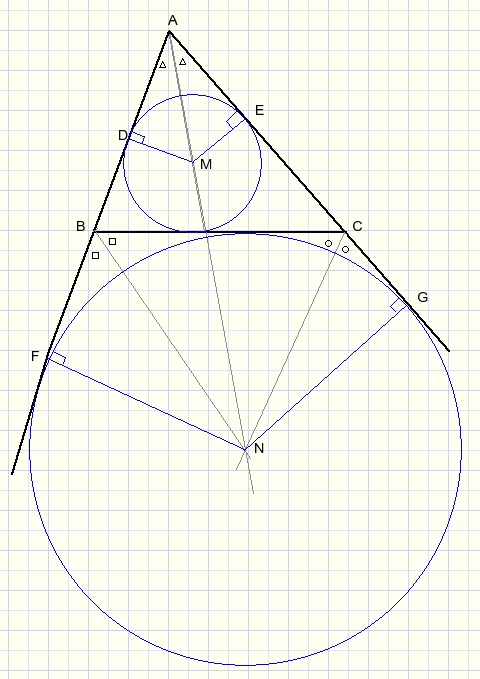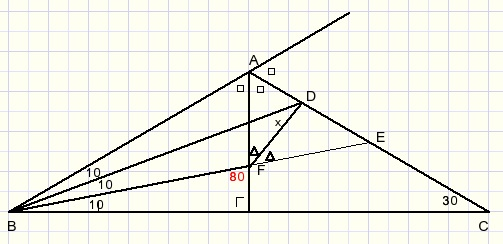geometry problem (8)Problem: calculate angle x in figure below:A great help is this lemma:
the exterior bisectors of two angles of a triangle and the bisector of the third angle intersect at one point.Please look at the picture above:
M is the intersection of the bisectors of ΔABC.
N is the intersection of the bisectors of exterior angles B and C.
M and N are the centers of the inscribed and escribed circles.

Lines DM and FN are both perpendicular to AB.
LAMD=LANF.
So, AN is a straight line.

The solution1. extend line BA
2. observe ΔABF
3. BD is interior bisector of B
3. AC is exterior bisector of A
4. D is the intersection of bisectors so, DF is exterior bisector of F
5. LAFE=800
6. LAFD=0,5*80=400
7. x+10 = 40
8. x = 300OneStat